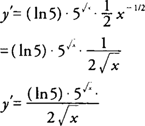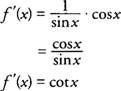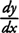## Differentiation of Exponential and Logarithmic Functions

Exponential functions and their corresponding inverse functions, called logarithmic functions, have the following differentiation formulas:Note that the exponential function f( x) = e x has the special property that its derivative is the function itself, f′( x) = e x = f( x).

Example 1: Find f′( x) ifExample 2: Find y′ if.Example 3: Find f′( x) if f( x) = 1n(sin x).Example 4: Findif y=log 10(4 x 2 − 3 x −5).